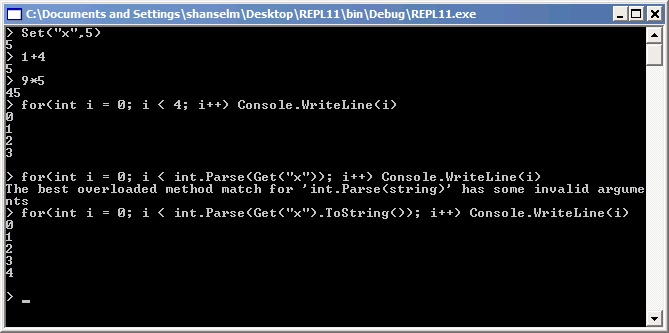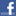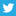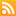# Scott Hanselman

## NotMuchofAREPL - CSREPL back-ported for .NET 1.1

May 27, '05 Comments  Posted in ProgrammingLately Don Box has been exploring just how dynamic a language C# can be.

Here's his REPL for C# code, with backward changes so you can play with it on .NET 1.1 since 1.1 doesn't have anonymous delegates.

I call it NotMuchOfAREPL. Diffs highlighted. I haven't run that many expressions through it so be warned. That said, it's pretty slick. There's a lot of untapped potential in the language (and that wouldn't require IL changes) that could be unlocked with a few new keywords. It's a very exciting time.

`    1 using System;`
`    2 using System.Reflection;`
`    3 using System.Text;`
`    4 using System.CodeDom.Compiler;`
`    5 `
`    6 namespace notmuchofarepl `
`    7 {`
`    8     class Program `
`    9     {`
`   10         static string funcPrefix = "using System;\r\n"`
`   11             + "public delegate void Proc();\r\n"`
`   12             + "public class Wrapper { \r\n"`
`   13             + "  public static object Set(string name, object value) { \r\n"`
`   14             + "    AppDomain.CurrentDomain.SetData(name, value);\r\n"`
`   15             + "    return value; \r\n"`
`   16             + "  }\r\n"`
`   17             + "  public static object Get(string name) { \r\n"`
`   18             + "    return AppDomain.CurrentDomain.GetData(name);\r\n"`
`   19             + "  }\r\n"`
`   20             + "  public static object Invoke(Proc proc) { \r\n"`
`   21             + "    proc();\r\n"`
`   22             + "    return null; \r\n"`
`   23             + "  }\r\n"`
`   24             + "  public static void notSoAnon() { \r\n";`
`   25         static string funcInter = "  ;"`
`   26             + "  }\r\n"`
`   27             + "  public static object Eval() { return ";`
`   28         static string funcSuffix = "; \r\n} }";`
`   29 `
`   30         static string StringEval(string expr, string voidExpr) `
`   31         {`
`   32             string program = funcPrefix + voidExpr + funcInter + expr + funcSuffix;`
`   33 `
`   34             ICodeCompiler compiler = new Microsoft.CSharp.CSharpCodeProvider().CreateCompiler();`
`   35 `
`   36             CompilerParameters cp = new CompilerParameters();`
`   37             cp.GenerateExecutable = false;`
`   38             cp.GenerateInMemory = true;`
`   39 `
`   40             CompilerResults results = compiler.CompileAssemblyFromSource(cp, program);`
`   41             if (results.Errors.HasErrors) `
`   42             {`
`   43                 if (results.Errors.ErrorNumber == "CS1525")`
`   44                     return StringEval("Invoke(new Proc(notSoAnon))",expr);`
`   45                 return results.Errors.ErrorText;`
`   46             }`
`   47             else `
`   48             {`
`   49                 Assembly assm = results.CompiledAssembly;`
`   50                 Type target = assm.GetType("Wrapper");`
`   51                 MethodInfo method = target.GetMethod("Eval");`
`   52                 object result = method.Invoke(null, null);`
`   53                 return result == null ? null : result.ToString();`
`   54             }`
`   55         }`
`   56 `
`   57         static void Main(string[] args) `
`   58         {`
`   59              while (true ) `
`   60             {`
`   61                 Console.Write("> ");`
`   62                 Console.Out.Flush();`
`   63                 string expr = Console.ReadLine();`
`   64                 if (expr == null)`
`   65                     break;`
`   66                 try `
`   67                 {`
`   68                     string result = StringEval(expr, String.Empty);`
`   69                     Console.WriteLine(result);`
`   70                 }`
`   71                 catch (TargetInvocationException ex) `
`   72                 {`
`   73                     Console.WriteLine(ex.InnerException.GetType().Name + ": " + ex.InnerException.Message);`
`   74                 }`
`   75                 catch (Exception ex) `
`   76                 {`
`   77                     Console.WriteLine(ex.GetType().Name + ": " + ex.Message);`
`   78                 }`
`   79             }`
`   80          }`
`   81     }`
`   82 }`